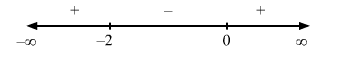Deepak Scored 45->99%ile with Bounce Back Crack Course. You can do it too!

# If the solve the problem

Question:

$f(x)=(x-1)(x+2)^{2}$

Solution:

Given: $f(x)=(x-1)(x+2)^{2}$

$\Rightarrow f^{\prime}(x)=(x+2)^{2}+2(x+2)(x-1)$

For a local maximum or a local minimum, we must have

$f^{\prime}(x)=0$

$\Rightarrow(x+2)^{2}+2(x+2)(x-1)=0$

$\Rightarrow(x+2)(x+2+2 x-2)=0$

$\Rightarrow(x+2)(3 x)=0$

$\Rightarrow x=0,-2$Since $f^{\prime}(x)$ changes from negative to positive when $x$ increases through $0, x=0$ is the point of local minima. The local minimum value of $f(x)$ at $x=0$ is given by

$(0-1)(0+2)^{2}=-4$

Since $f^{\prime}(x)$ changes sign from positive to negative when $x$ increases through $-2, x=-2$ is the point of local maxima. The local maximum value of $f(x)$ at $x=-2$ is given by

$(-2-1)(-2+2)^{2}=0$